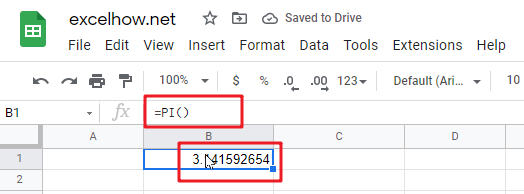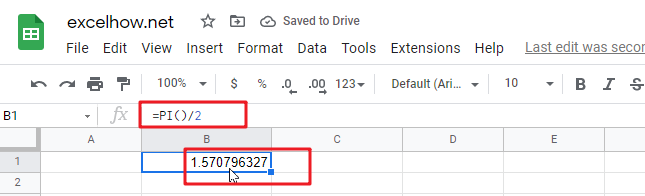# ExcelHow

This post will guide you how to use Google Sheets PI function with syntax and examples in Google Spreadsheets.

## Description

The Google Sheets PI function returns the value of mathematical constant PI. The returned value is 3.14159265358979 and ti will accurate to 15 digits.

The PI function is a build-in function in Google Spreadsheets and it is categorized as a Math and Trigonometry Function.

## Syntax

The syntax of the PI function is as below:

`=PI()`

The PI function has no any arguments.

## Google Sheets PI Function Examples

The below examples will show you how to use Google Sheets PI Function to return the value of PI.

1# get the value of PI, enter the following formula in Cell B1.

`=PI()`2# get the value of PI divided by 2, enter the following formula in Cell B2.

`=PI()/2`